# 8+ Best Free Monthly Budget Template

This article will show you some free and printable personal monthly budget templates or family monthly budgets for google sheet and Microsoft Excel spreadsheet applications, and will also explain some of the features or important functions of these templates. This will make it easy for you to make modifications when using these templates so that you can customize the monthly budget template to meet your specific requirements.

If your personal income or household income is calculated on a weekly basis, then you can also use these spreadsheet budget templates as weekly budget templates.

## What Is A Monthly Budget?

A monthly budget is a budget planning used for an individual or household’s monthly income and monthly expenses to determine the balance of monthly savings or debts. A monthly budget is not much different from a personal or household budget, it just specifies the time frame for the budget.

A monthly budget is a plan that you create for each month based on your personal or household income and expenses. This is a plan for all of your expense amounts, which also includes funds for recreational activities or personal entertainment expenses, so creating a solid budget plan can help you achieve your financial goals.

A budget planning can include a comprehensive list of income or expenses, and you can focus on several categories. You can write a budget plan manually or design a reliable, printable monthly budget spreadsheet by using Google Sheets or Microsoft Excel Spreadsheet.

## Why Is A Monthly Budget Important?

A simple budget planning is a great benefit for every person or family, not just for those who are struggling financially at the moment. A budget plan encourages you to use your spending on sensible spending items.

It encourages you to live within your means and to put your money to work in the best way possible. Having a designated budget plan is one way to achieve your financial goals. It can help you：

• Track your income and expenses, so you know exactly how much you are saving or spending each month
• Help you plan ahead to use available funds for emergency aggressive or savings goals in preparation for future retirement.
•  Relieves financial stress and good planning can help you have a healthy financial situation

## What Is A Monthly Budget Template?

A monthly budget template is a tool to help you quickly create a personal or household monthly budget. The budget template will contain two separate columns for monthly income or expenses, and each column will contain specific income items or expense items.

## 1. Personal Monthly Budget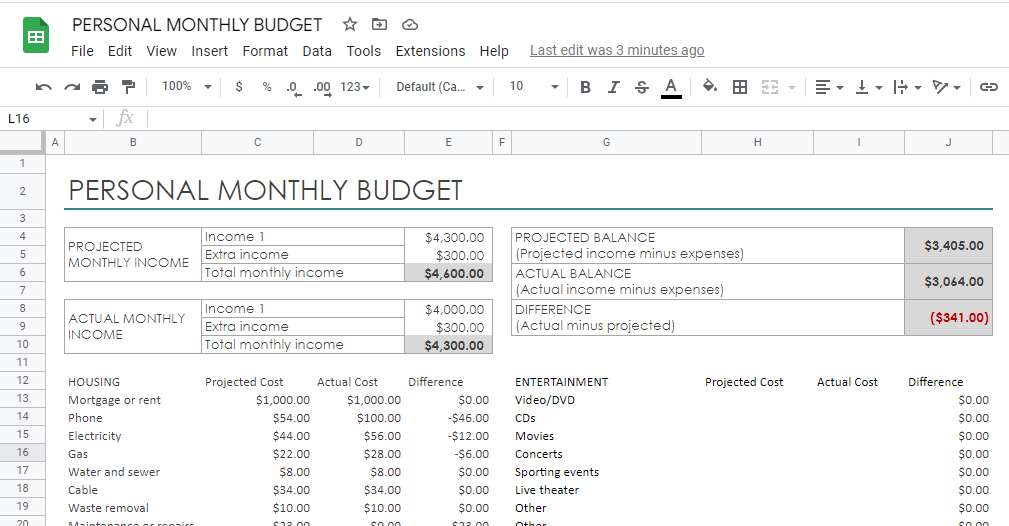This template is an official personal monthly budget template provided by Microsoft Excel, if you need to use this template in google sheets, then you just need to import the budget template file into google sheets.

You can use this personal monthly budget worksheet to track your monthly total projected income or total actual income and total projected expenses and total actual costs.

You just need to enter various categories of expenses in the form and the projected balance, actual balance and difference will be calculated automatically.

Let’s take a look at how to use this personal monthly budget template.

STEP 1: Firstly, you need to enter your total projected monthly income, which includes the main income item Income 1 and the Extra income item. After that, the total projected monthly income will be calculated automatically by using the SUM formula. The SUM formula used is as follows.

`=SUM(E4:E5)`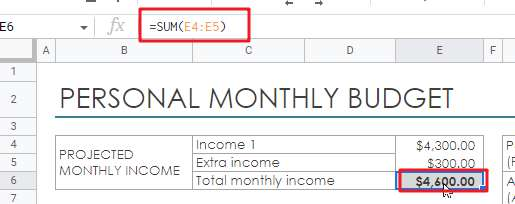As you can see from the above figure, the SUM formula will automatically calculate the sum of Income1 and Extra income.

STEP 2: You need to enter your actual monthly income in cell E8, which contains the actual income of the month for the main income items Income 1 and Extra income.

When you have entered Income 1 and Extra income, the actual monthly income for that month will also be calculated automatically by the SUM function. The formula used is as follows.

`=SUM(E8:E9)`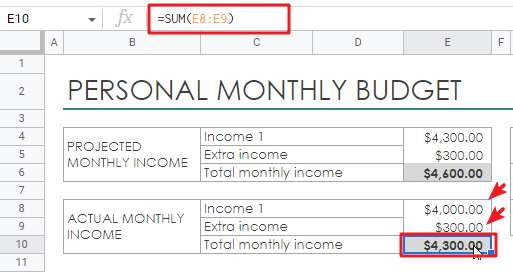STEP 3: You need to enter the projected costs and actual costs incurred on various categories in the corresponding cells. The template contains 12 major expense categories: HOUSING, TRANSPORTATION, INSURANCE, FOOD, PETS, PERSONAL CARE, ENTERTAINMENT, LOANS, TAXES, SAVINGS OR INVESTMENTS, GIFTS AND DONATIONS and LEGAL.

When you enter the projected cost and the actual cost, the difference between the two costs will also be automatically calculated by the function. The formula is as follows.

`='PERSONAL MONTHLY BUDGET'!\$C14-'PERSONAL MONTHLY BUDGET'!\$D14`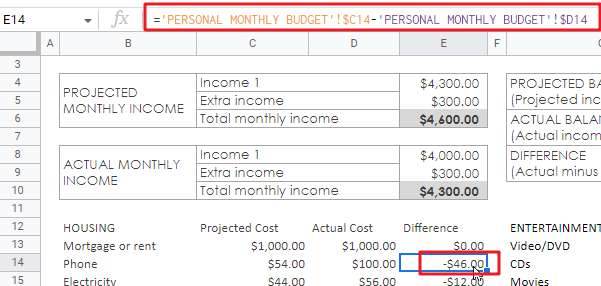STEP 4: Once the projected and actual costs for all categories are entered into the monthly budget worksheet, this template will automatically do a lot of statistical tasks, such as PROJECTED BALANCE, ACTUAL BALANCE, DIFFERENCE, TOTAL PROJECTED COST, TOTAL ACTUAL COST , TOTAL DIFFERENCE.

TOTAL PROJECTED COST

The TOTAL PROJECTED COST counts the total projected cost of all expenditure items and is calculated using the following SUBTOTAL formula.

`=SUBTOTAL(109,'PERSONAL MONTHLY BUDGET'!\$C\$13:\$C\$22,'PERSONAL MONTHLY BUDGET'!\$C\$26:\$C\$32,'PERSONAL MONTHLY BUDGET'!\$C\$36:\$C\$39,'PERSONAL MONTHLY BUDGET'!\$C\$43:\$C\$45,'PERSONAL MONTHLY BUDGET'!\$C\$49:\$C\$53,'PERSONAL MONTHLY BUDGET'!\$C\$57:\$C\$63,'PERSONAL MONTHLY BUDGET'!\$H\$13:\$H\$21,'PERSONAL MONTHLY BUDGET'!\$H\$25:\$H\$30,'PERSONAL MONTHLY BUDGET'!\$H\$34:\$H\$37,'PERSONAL MONTHLY BUDGET'!\$H\$41:\$H\$43,'PERSONAL MONTHLY BUDGET'!\$H\$47:\$H\$49,'PERSONAL MONTHLY BUDGET'!\$H\$53:\$H\$56)`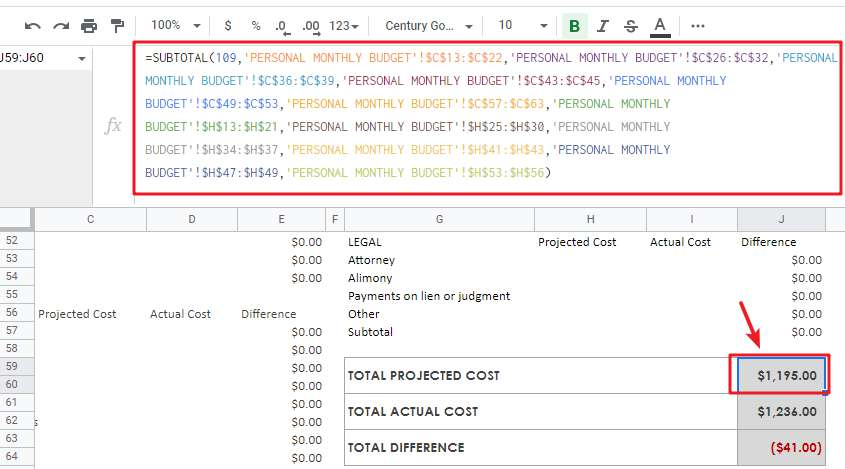TOTAL ACTUAL COST

The TOTAL ACTUAL COST is used to calculate the total actual cost of all expense items, which is summarized by the following SUBTOTAL formula.

`=SUBTOTAL(109,'PERSONAL MONTHLY BUDGET'!\$D\$13:\$D\$22,'PERSONAL MONTHLY BUDGET'!\$D\$26:\$D\$32,'PERSONAL MONTHLY BUDGET'!\$D\$36:\$D\$39,'PERSONAL MONTHLY BUDGET'!\$D\$43:\$D\$45,'PERSONAL MONTHLY BUDGET'!\$D\$49:\$D\$53,'PERSONAL MONTHLY BUDGET'!\$D\$57:\$D\$63,'PERSONAL MONTHLY BUDGET'!\$I\$13:\$I\$21,'PERSONAL MONTHLY BUDGET'!\$I\$25:\$I\$30,'PERSONAL MONTHLY BUDGET'!\$I\$34:\$I\$37,'PERSONAL MONTHLY BUDGET'!\$I\$41:\$I\$43,'PERSONAL MONTHLY BUDGET'!\$I\$47:\$I\$49,'PERSONAL MONTHLY BUDGET'!\$I\$53:\$I\$56)`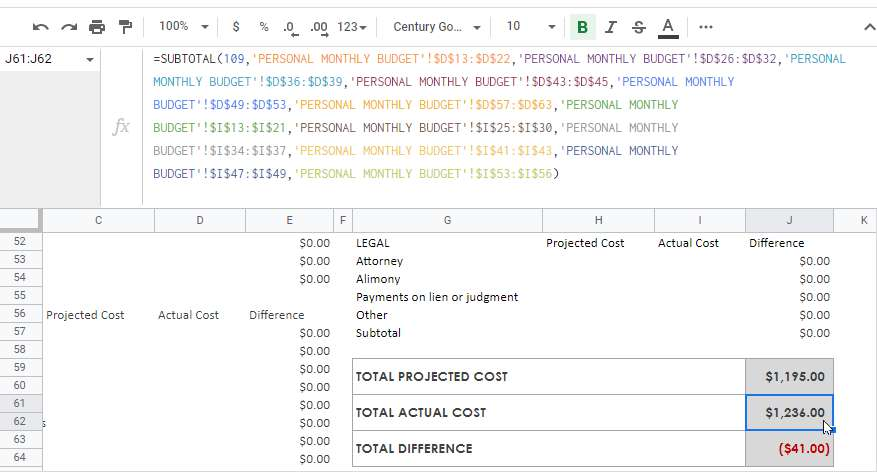PROJECTED BALANCE:

PROJECTED BALANCE is used to calculate the total projected income minus the total projected cost. The total projected cost is calculated using the formula above.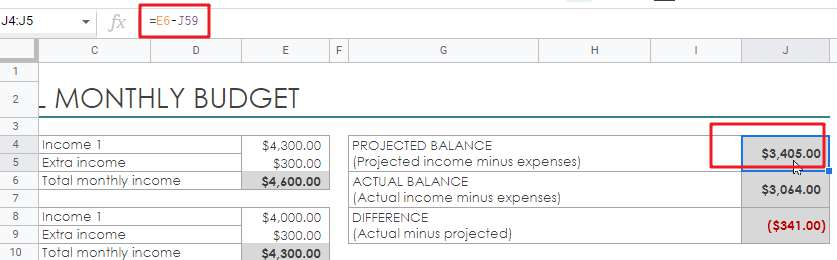ACTUAL BALANCE

ACTUAL BALANCE is used to calculate the total actual income minus the actual cost. Where the total actual cost is calculated using the formula above.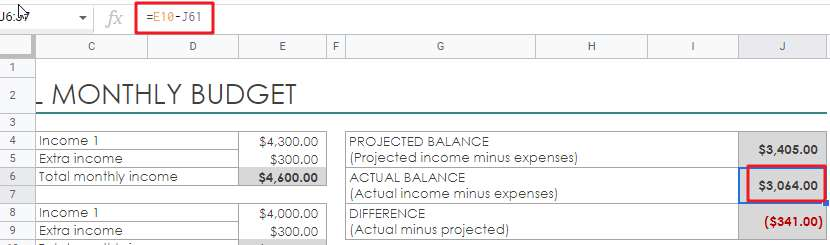If you have other requirements for a customized budget template, you can send us a message.

If you are looking for personal monthly budget template, check out Microsoft office site by clicking here.

## 2. Simple Monthly Budget Template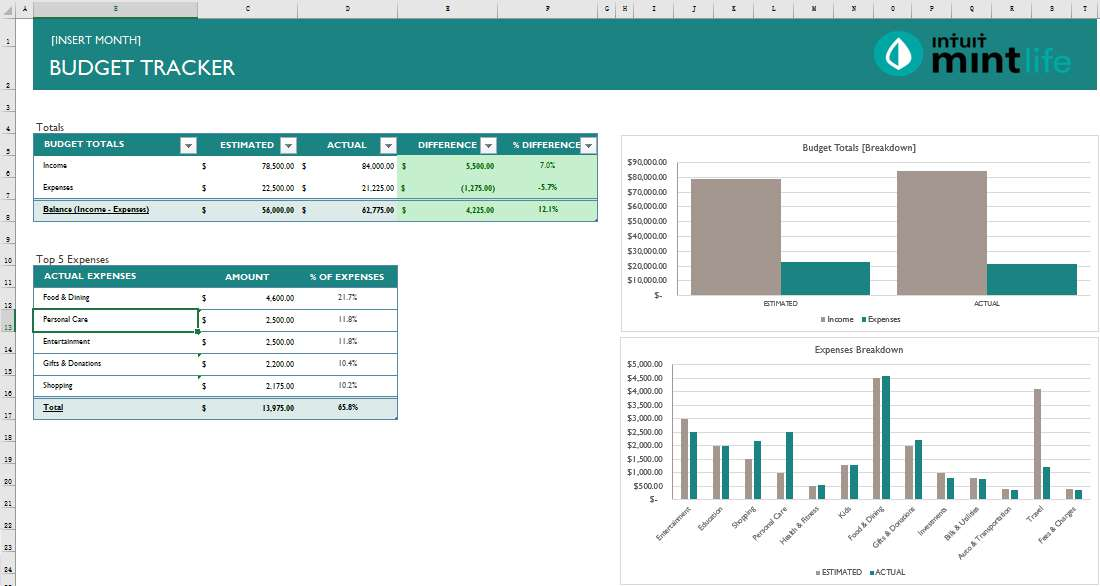This template is designed by MintLife and it needs to be opened by default via Microsoft Excel Spreadsheet.

Note: You cannot use this monthly budget template in google sheets because many of the statistical formulas in the template are only available in Microsoft Excel and google sheets does not recognize these formulas. If you are willing to use this template in google sheets, you can leave us a comment and we will help you how to adapt these formulas in google sheets.

The monthly budget template contains three parts: Income Input, Expense Input, Budget Summary. These three parts are placed in three separate worksheets.

Let’s see how to use this monthly budget worksheet:

STEP1: First you need to go to the Income Inputs worksheet and enter the estimated income and actual income in the corresponding income items under the ESTIMATED and ACTUAL columns. You will then see that the value of the income difference will be automatically calculated using Microsoft Excel or Google Sheets formulas.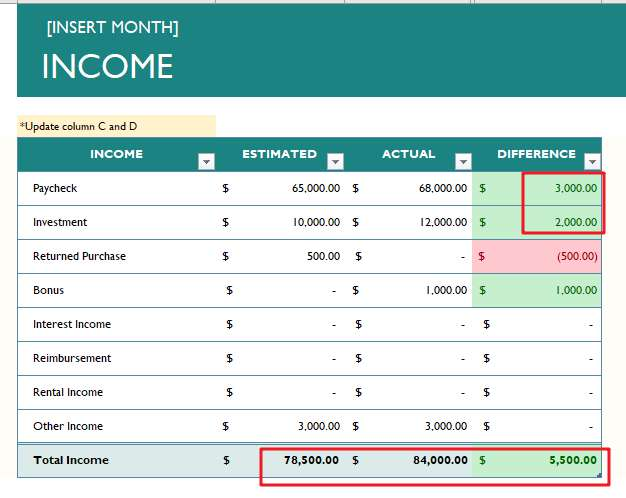As you can see from the above figure, the total projected income and the total actual income are also counted automatically.

The formula to count the projected total income is as follows.

`=SUBTOTAL(109,[ESTIMATED])`

The formula to count the actual total income is as follows.

`=SUBTOTAL(109,[ACTUAL])`

Both projected and actual total incomes need to be summed up by the SUBTOTAL function for the corresponding ESTIMATED and ACTUAL columns.

STEP2: In the Expense Inputs worksheet, you need to enter the estimated and actual costs for the corresponding expense items in the ESTIMATED and ACTUAL columns. The values in the DIFFERENCE column will be automatically calculated.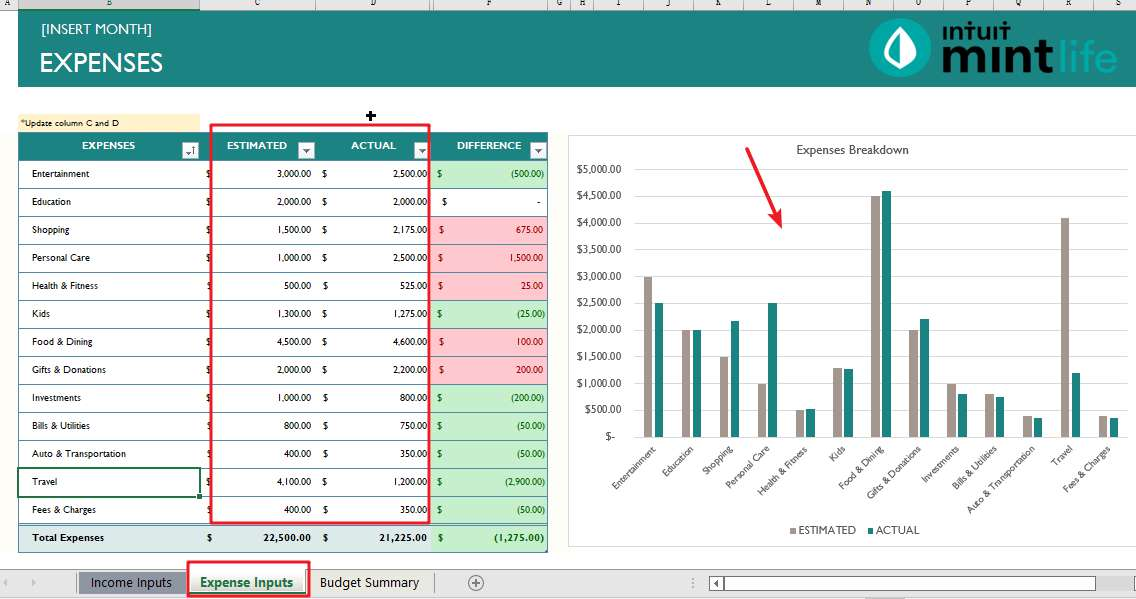Once all the expense items are entered, the total projected expense and total actual cost values are also automatically calculated. This template calculates the total expenses by using the SUBTOTAL function and automatically calculates the difference between the estimated total expenses and the actual total cost.

The formula is as follows.

```=SUBTOTAL(109,[ESTIMATED])
=SUBTOTAL(109,[ACTUAL])
=SUBTOTAL(109,[DIFFERENCE])```

From the above figure, we can also see that the Expense inputs worksheet has also added a Clustered Column chart, so you can see more clearly the ratio of estimated and actual expenses for each item.

STEP3: After entering the Income Inputs and Expense Inputs worksheets, you can go to the Budget Summary worksheet.

This worksheet contains 2 statistics, one for total income and total expenses, and one for the top 5 actual expenses.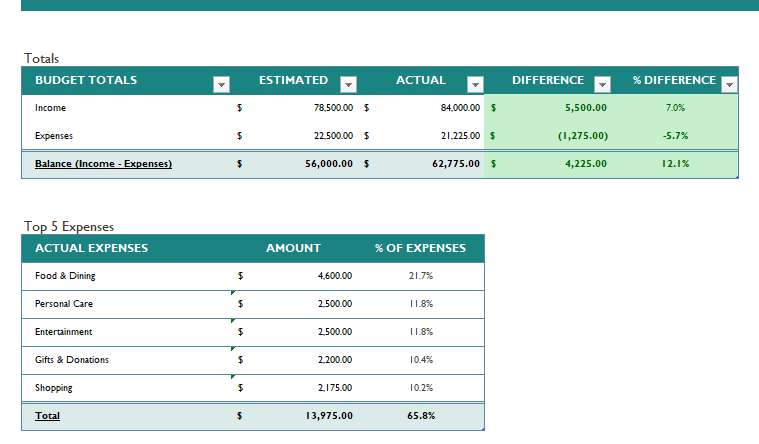As you can see from the above screenshot, Total Projected Income, Total Actual Income, Total Projected Expenses, and Total Actual Cost are automatically extracted from the Income Inputs and Expense Inputs worksheets using the following formulas.

```=Income[[#Totals],[ESTIMATED]]

=Income[[#Totals],[ACTUAL]]

=Expenses[[#Totals],[ESTIMATED]]

=Expenses[[#Totals],[ACTUAL]]```

Where Income and Expenses are the two defined Name Ranges.

```Income = "Income Inputs"! \$B\$6:\$F\$13

Expenses = "Expense Inputs"! \$B\$6:\$F\$18```

This template will count the top five actual expense items and automatically calculate the percentage of each expense item to the total expense amount. The top five spend items are found from the Expense Inputs table using the following LARGET function.

`=LARGE(Expenses[TOP 5 AMOUNT],1)`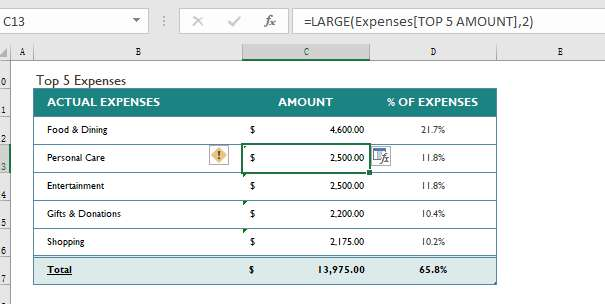If you are looking for simple monthly budget template, check out mint site by clicking here.

## 3. Detailed Monthly Budget Template For Excel Sheet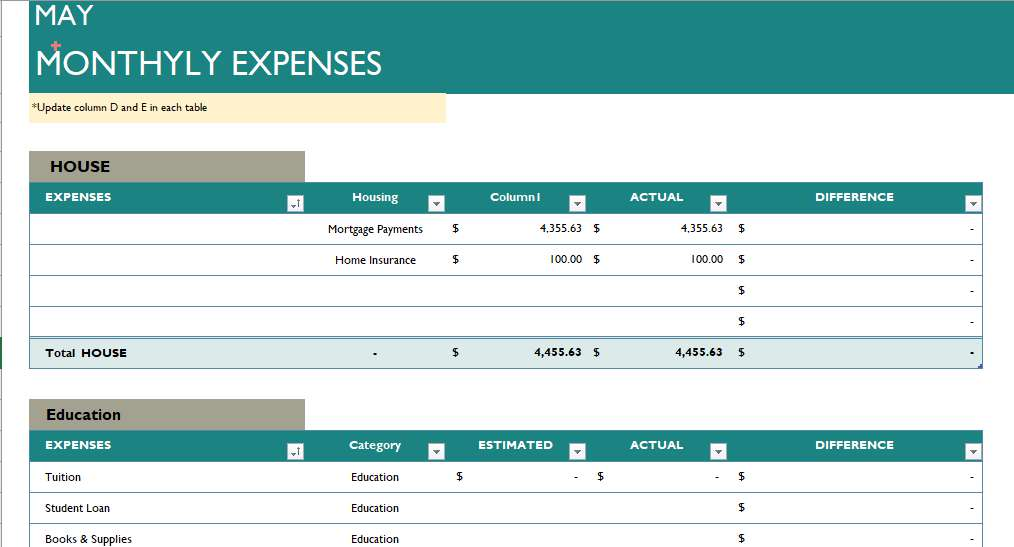This template is also designed by MintLife and needs to be opened and used by Microsoft Excel Spreadsheet by default.

The difference between this template and the simple monthly budget template above is that the Expense Inputs worksheet categorizes all expense items and contains 12 expense categories: House, Education, Shopping, Health & Fitness, Personal Care, Kids, Food & Dining, Gifts & Donations, Investment, Bills & Utilities, Auto & Transportation, Travel and Fees & Charges. Dining, Gifts & Donations, Investment, Bills & Utilities, Auto & Transportation, Travel and Fees & Charges.

Note: You cannot use this monthly budget template in google sheets because many of the statistical formulas in the template are only available in Microsoft Excel and google sheets does not recognize these formulas. If you are interested in using this template in google sheets, you can send us a message and we will assist you on how to adapt these formulas in google sheets.

This template is used in the same way as the previous one, when you have finished entering the income and expense items, the Budget Summary page will also have separate statistics based on expense categories. The following VLOOKUP formula is used to get the estimated and actual total expenses for each expense category.

```=IFERROR(VLOOKUP([@[EXPENSE CATEGORIES]],'Expense Inputs'!B:F,3,FALSE),"-")

=IFERROR(VLOOKUP([@[EXPENSE CATEGORIES]],'Expense Inputs'!B:F,4,FALSE),"-")```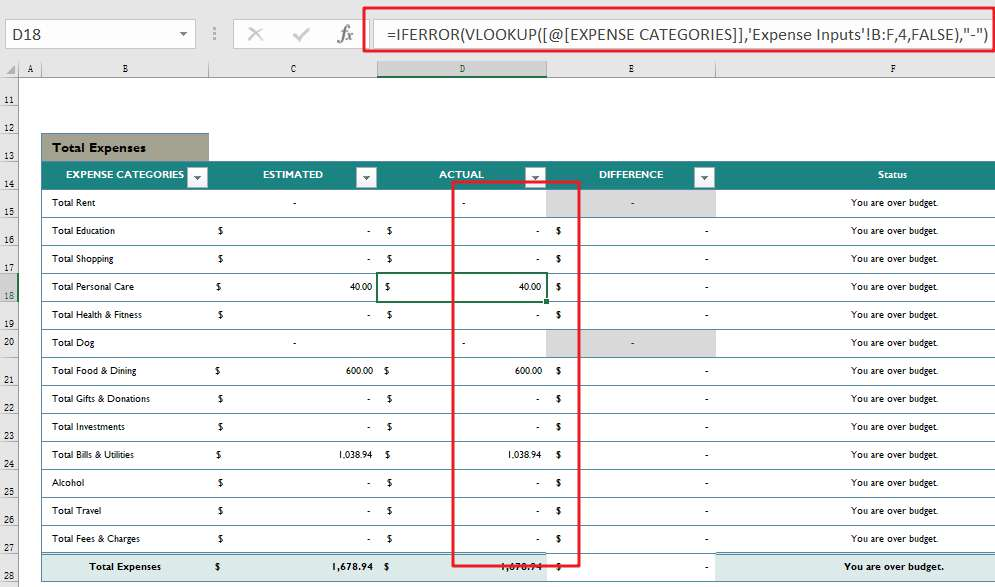The total expense table also automatically calculates the difference for each expense category and automatically determines whether the current category exceeds the budgeted amount based on the difference.

If you are looking for a detailed monthly budget template, check out mint site by clicking here.

## 4. Family Monthly Budget Planner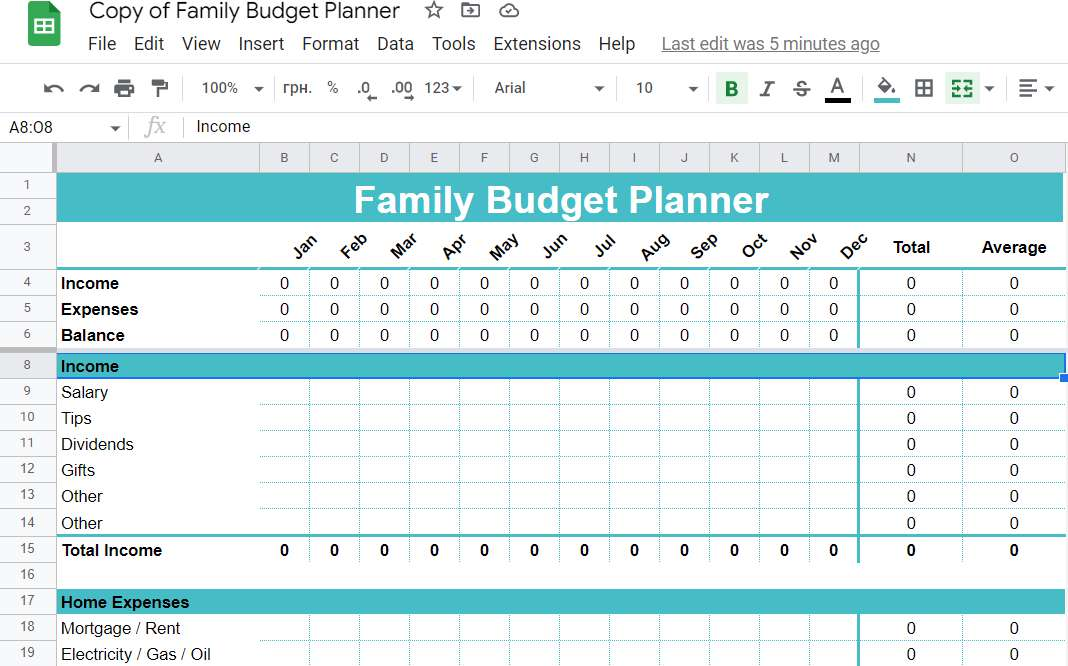This template is a free and printable blank monthly household budget template that you will need to open it via google sheets. If you need to use it in Microsoft Excel Spreadsheets, then you will need to download it as a local xls file via google sheets.

The template freezes the first six rows so that the income, expense and balance information for each month is always displayed. The first six rows contain the total family income, total family expenses and total balance for each month, and the total family income and total family expenses for a year are also calculated automatically.

The formulas used are as follows.

`=sum(B4:M4)`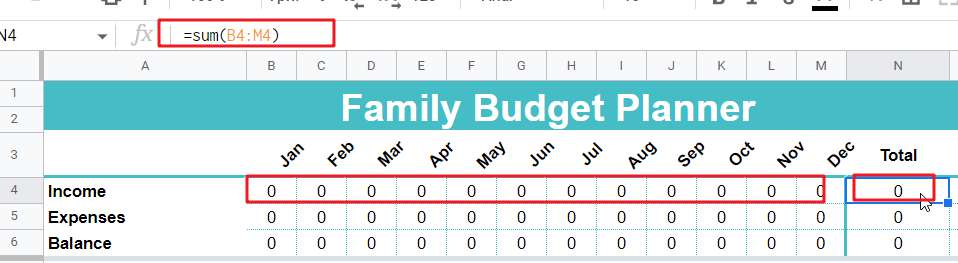Let’s see how to use this monthly household budget template.

STEP1: First you need to enter the values of the various income items in the corresponding month column in the Income table.STEP2: You need to enter the actual expenses in the expense category sub-table; after that you will find that the relevant statistical items in the template will be calculated automatically.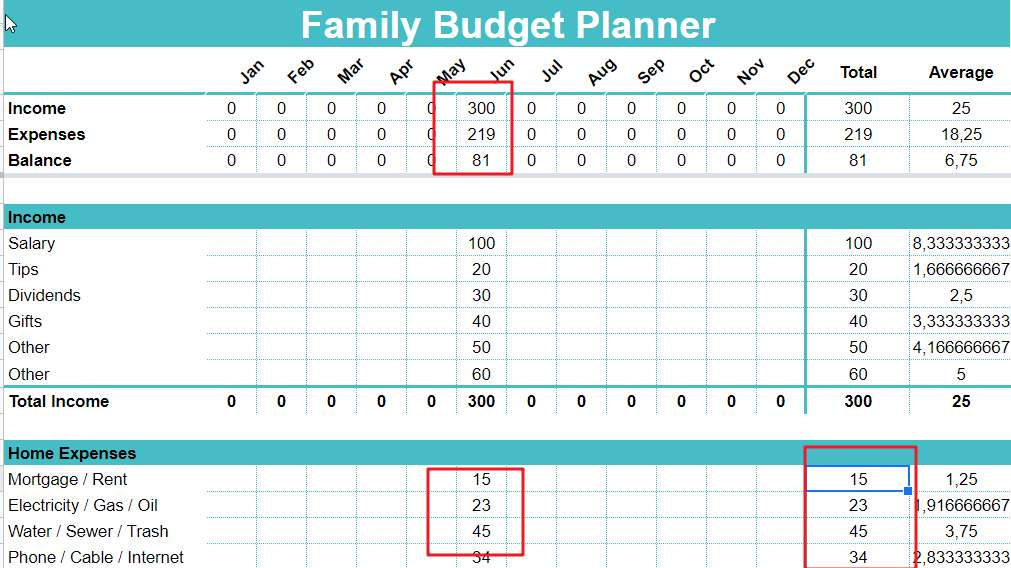This template contains only actual income items and expense items, and does not include projected income and projected expense.

If you are looking for a blank family monthly budget template, check out it by clicking here.

## 5. Personal Monthly Budget Template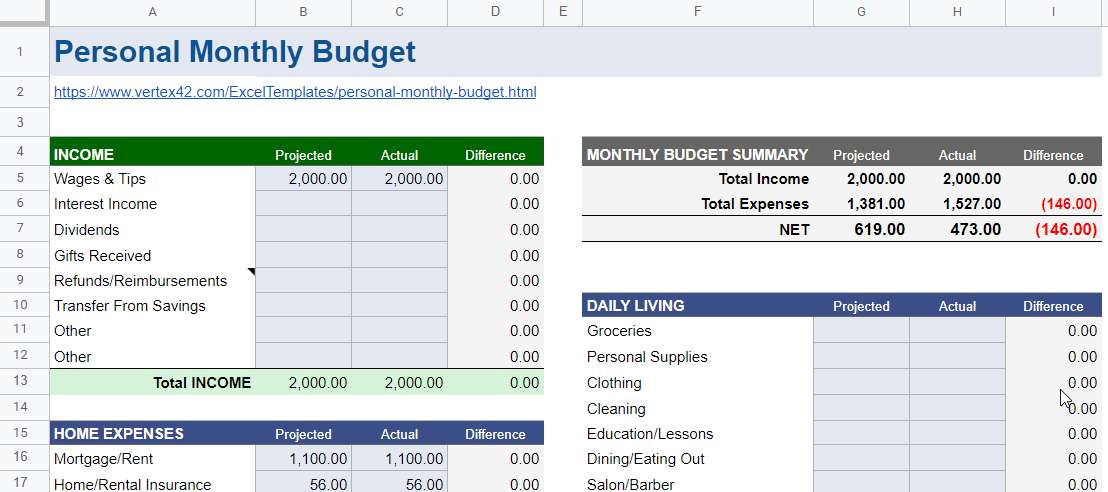This template is designed by Vertex42 and this template needs to be opened by Google sheets by default.

This free monthly personal budget template includes an income breakdown, an expense breakdown, and a monthly budget summary.

The income table contains 3 parts: projected income, actual income and income difference.

The expense table is recorded by different expense categories and also contains 3 parts: Estimated Expenses, Actual Expenses, and Expense Difference. The table contains ten expense categories: HOME EXPENSES, TRANSPORTATION, HEALTH, CHARITY/GIFTS, SUBSCRIPTIONS, SAVINGS, OBLIGATIONS and MISCELLANEOUS.

The usage of this template is basically the same as the first template, you just need to enter the corresponding data in the INCOME and EXPENSES tables, and the various statistics will be calculated automatically.

The MONTHLY BUDGET SUMMARY table will automatically calculate the total projected income, total actual income, total projected expenses and total actual expenses.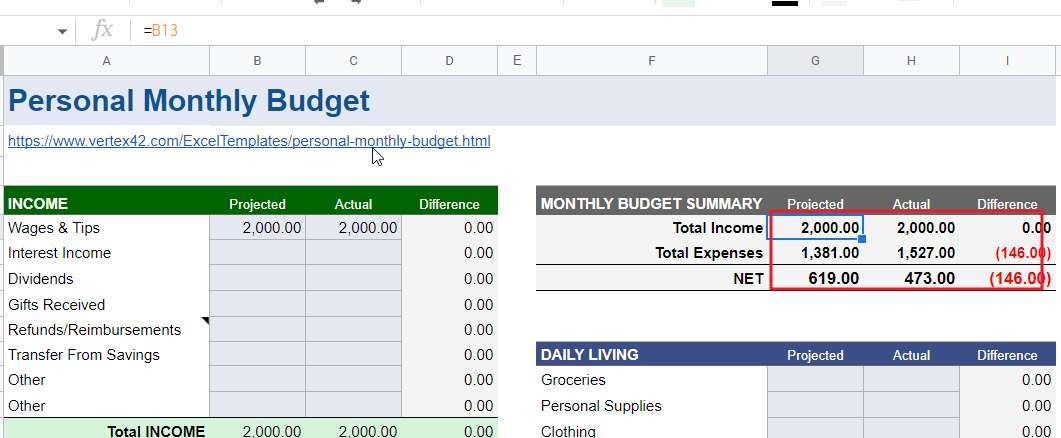If you are looking for a personal monthly budget template, check out it by clicking here.

## 6. Personal Monthly Budget Template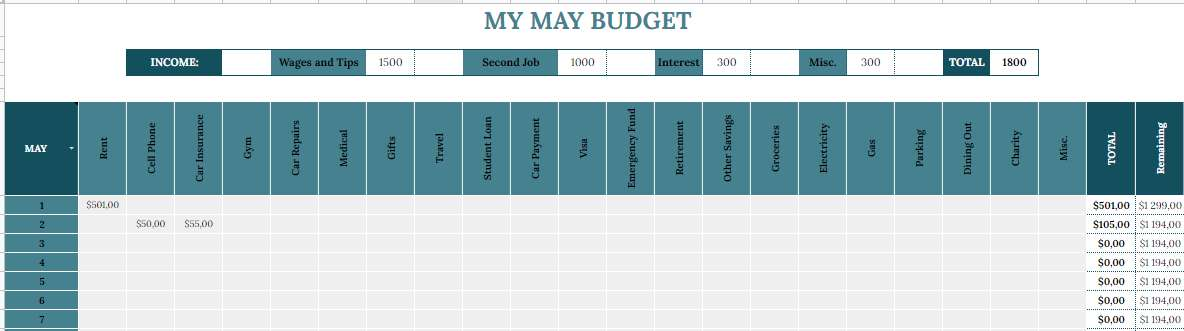This is a very specific personal monthly budget template. This template contains two parts: income breakdown and expense breakdown. This template will count the expenses for each day of the given month.

Let’s see how to use this monthly household budget template.

STEP1: You need to enter the various actual income values in the INCOME line and the total actual income will be calculated automatically.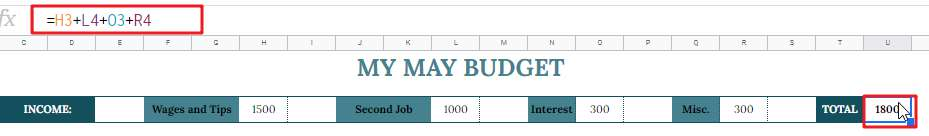STEP2: In the expense table, select the current month, for example, select May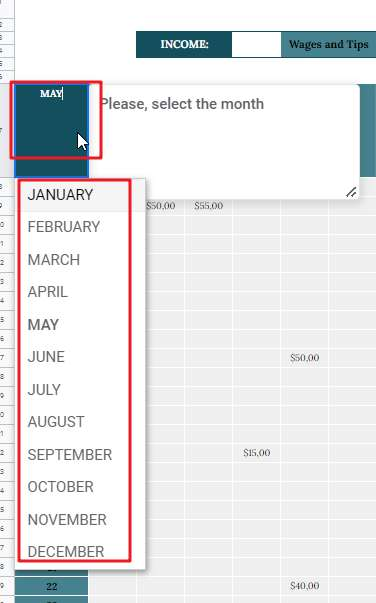STEP3:  After that you need to enter your actual daily expenses in the expenses tab. If your expense items are not shown in the expense table, then you can add them by yourself.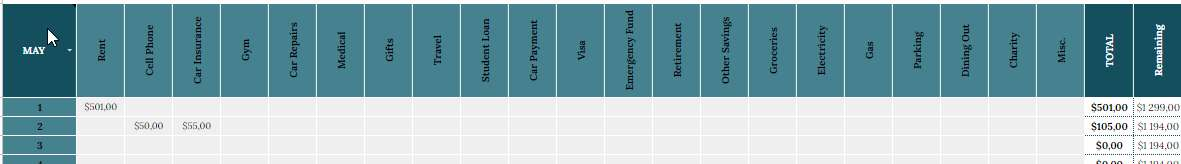After that, the total expenses and the current available balance for the day will be calculated. The formula used for this is as follows.

```=SUM(B8:V8)

=X8-sum(B9:V9)```

STEP4: The template also calculates the actual expense for a given month on an expense item, and will automatically calculate the difference.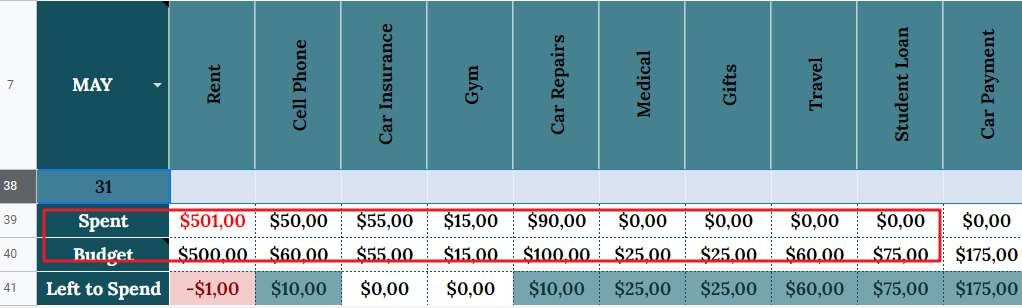If you are looking for a personal monthly budget template, check out it by clicking here.

## 7. Template Business Monthly Budget Template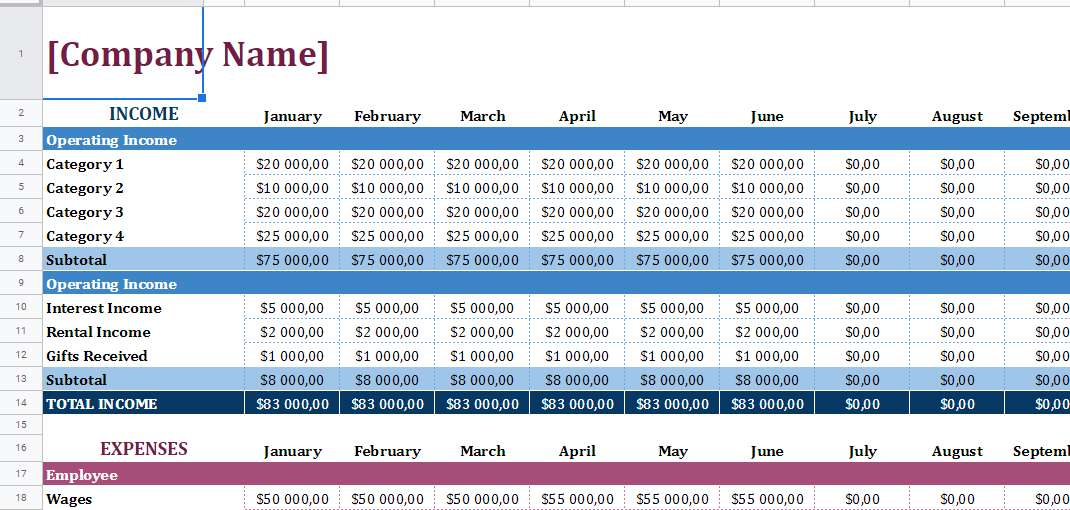This template is a business monthly budget template that counts the monthly revenue and various expense items of the company for each month.

The template categorizes the company’s revenue items and contains various sub-categories under each income category, and counts the actual revenue of each sub-category for each month.

The template also categorizes the company’s expense items, and contains various expense items under each expense category.

This template summarizes all revenue items and all expense items for each month.

Let’s see how to use this template:

STEP1: Enter the name of your company and insert the company logo

STEP2: You need to define the income categories and income subheadings according to your company and enter the actual income values for each month. When you entered all the revenue item values for a certain month, you can see that the total revenue for that month will also be counted automatically. The formula used is as follows.

`=ROUND(C8+C13;2)`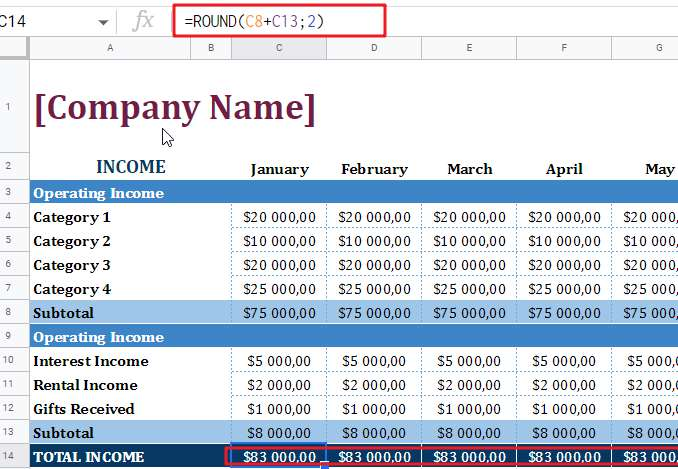When the value of the income items for certain months is entered, you should see that the total income for the year is also automatically calculated;

The following formula is used：

`=ROUND(O8+O13;2)`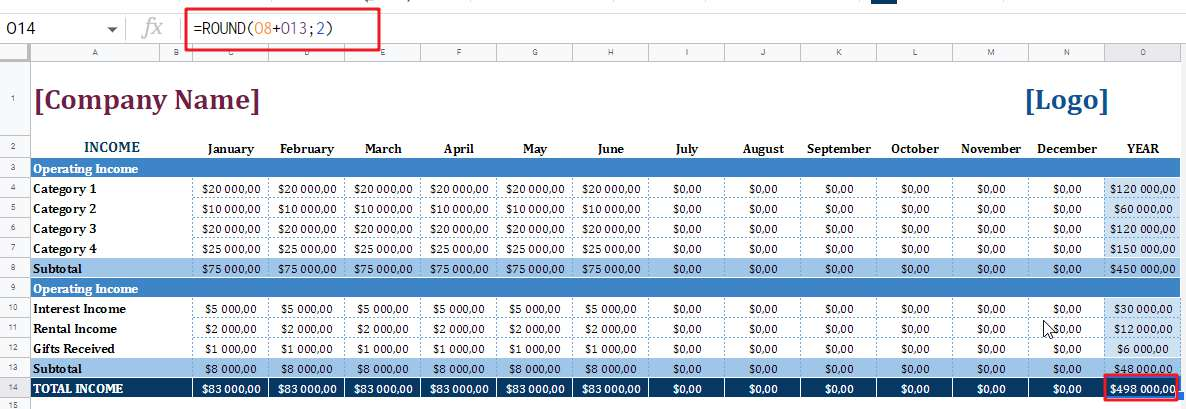STEP 4: Enter the actual expenses in each month’s expense item, and the total expenses for each month will also be calculated automatically. When the actual expenses are entered in the expense items of multiple months, the actual expenses of the year will also be calculated.

`=round(O20+O30+O36+O40;2)`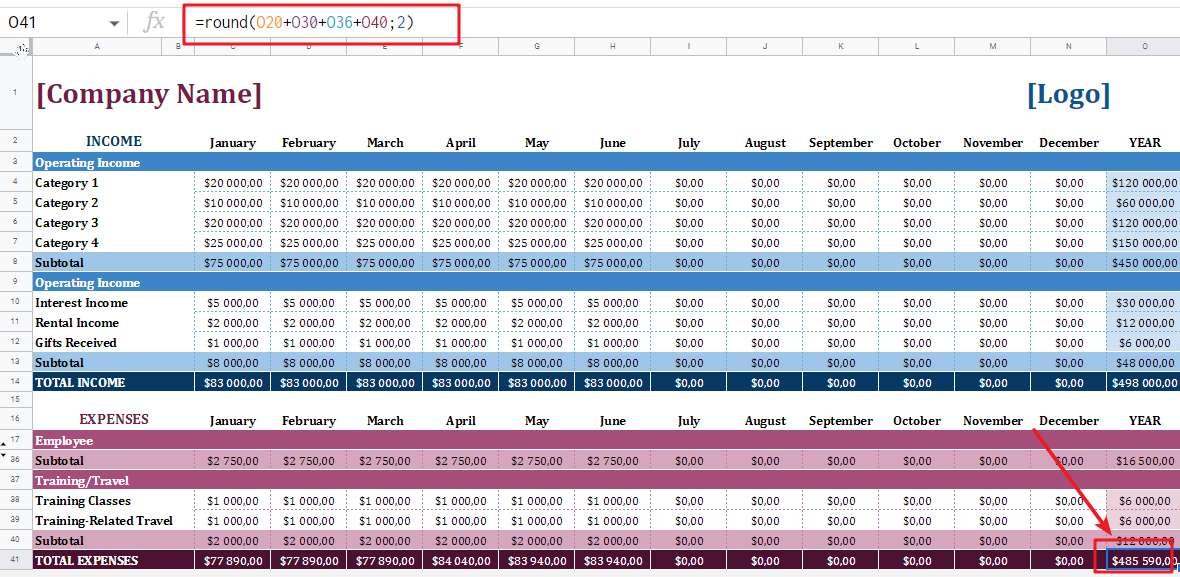If you are looking for a free business monthly budget template, check out it by clicking here.

## 8. Monthly Budget Template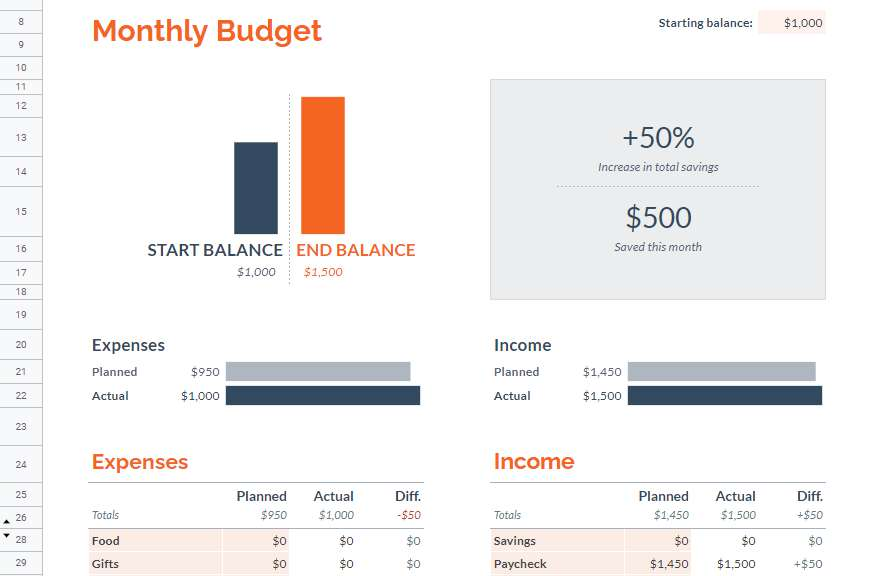This monthly budget template is officially provided by google sheets, where the Summary worksheet contains various statistical functions.

Let’s see how to use this template:

STEP 1: First of all, you need to set your starting budget balance in cell L8 of the summary sheet.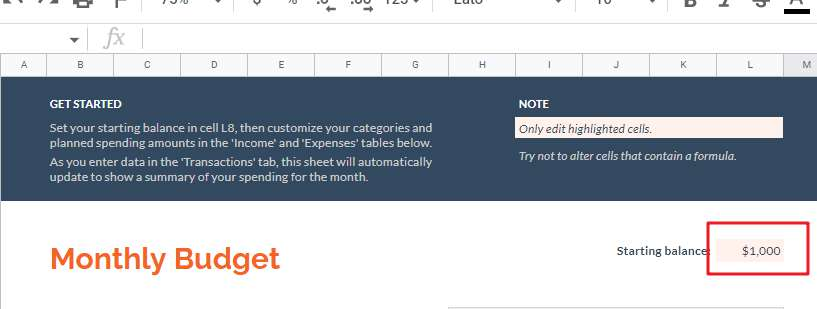STEP2: Customize your categories and planned expense amounts in the Income and Expenses table of the Summary worksheet.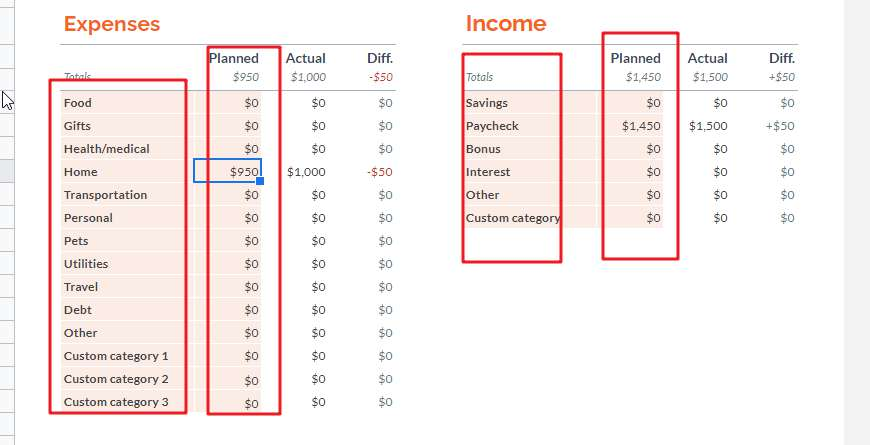STEP 3: Enter the expense and income data in the transaction worksheet, after that you will see the actual income data and the actual expense data in the income and expense tables will be updated automatically in the summary worksheet as well.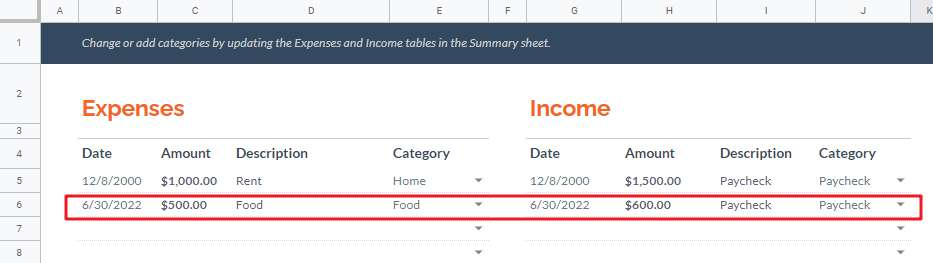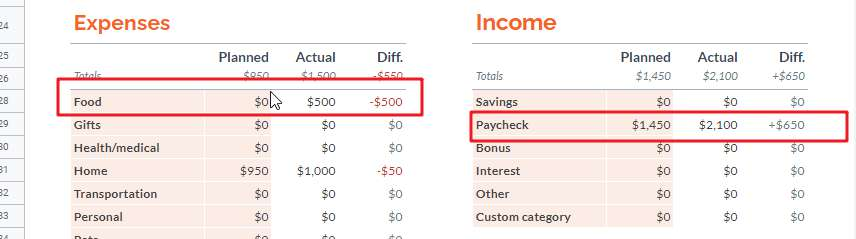STEP 4: This budget template shows projected total expenses, actual total expenses, projected total income and actual total income in the form of small charts, allowing us to visualize the status of variances.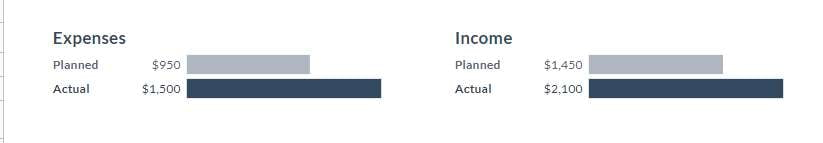If you are looking for a free business monthly budget template, check out it by clicking here.

### Related Functions

• Excel SUM function
The Excel SUM function will adds all numbers in a range of cells and returns the sum of these values. You can add individual values, cell references or ranges in excel.The syntax of the SUM function is as below:= SUM(number1,[number2],…)…